## Free Statistics

of Irreproducible Research!

Author's title
Author*Unverified author*
R Software Modulerwasp_meanplot.wasp
Title produced by softwareMean Plot
Date of computationFri, 22 Oct 2010 09:32:09 +0000
Cite this page as followsStatistical Computations at FreeStatistics.org, Office for Research Development and Education, URL https://freestatistics.org/blog/index.php?v=date/2010/Oct/22/t1287739959ulvvd67g79eiyeh.htm/, Retrieved Tue, 05 Dec 2023 21:31:23 +0000
Statistical Computations at FreeStatistics.org, Office for Research Development and Education, URL https://freestatistics.org/blog/index.php?pk=87353, Retrieved Tue, 05 Dec 2023 21:31:23 +0000
QR Codes:Original text written by user:
IsPrivate?No (this computation is public)
User-defined keywordsKDGP1W52
Estimated Impact134
Family? (F = Feedback message, R = changed R code, M = changed R Module, P = changed Parameters, D = changed Data)
-     [] [Centrummaten Maxi...] [1970-01-01 00:00:00] [4c9a292db13b4ee392c67e5938709d2c]
- RM D  [Central Tendency] [centrummaten eige...] [2010-10-20 11:01:39] [4c9a292db13b4ee392c67e5938709d2c]
- RMPD      [Mean Plot] [Eigen reeks seizo...] [2010-10-22 09:32:09] [c6c4f641f0c0971fe864266cb6b6a9b0] [Current]
Feedback Forum

Post a new message
Dataseries X:
100.4
97.7
97
96.5
98.4
106.3
103.1
102.4
95
98.1
106.1
99.1
101.2
95.5
99.8
97.1
97.5
96.8
97.7
100.9
94.3
99.5
100.8
97
99.2
101
102.3
97
91.2
97.6
95.7
100.5
94.4
102.9
105.1
98.8
100.7
99.6
107.7
102.9
101.6
102.7
110.5
109.8
94.3
102.5
105
102.3
107.7
100.3
99.5
95
97.7
96.3
97.8
106.4
96.1
106.2
114.7
111.9
121
117.7
115.4
114.3
109.5
108.1
108.2
99.1
101.2
98.1
95.5
97.9
98.2
98.7
95.6
95.8
94.4
96.5
103.3
104.3
104.5
102.3
103.8
103.1
102.2
106.3
102.1
94
102.6
102.6
106.7
107.9
109.3
105.9
109.1
108.5
111.7
109.8
109.1
108.5
108.5
106.2
117.1
109.8
115.2
115.9
119.2
121
118.6
117.6
114.6
110.6
102.5
101.6
107.4
105.8
102.8
104
100.4
100.6

 Summary of computational transaction Raw Input view raw input (R code) Raw Output view raw output of R engine Computing time 6 seconds R Server 'Gwilym Jenkins' @ 72.249.127.135

\begin{tabular}{lllllllll}
\hline
Summary of computational transaction \tabularnewline
Raw Input & view raw input (R code)  \tabularnewline
Raw Output & view raw output of R engine  \tabularnewline
Computing time & 6 seconds \tabularnewline
R Server & 'Gwilym Jenkins' @ 72.249.127.135 \tabularnewline
\hline
\end{tabular}
%Source: https://freestatistics.org/blog/index.php?pk=87353&T=0

[TABLE]
[ROW][C]Summary of computational transaction[/C][/ROW]
[ROW][C]Raw Input[/C][C]view raw input (R code) [/C][/ROW]
[ROW][C]Raw Output[/C][C]view raw output of R engine [/C][/ROW]
[ROW][C]Computing time[/C][C]6 seconds[/C][/ROW]
[ROW][C]R Server[/C][C]'Gwilym Jenkins' @ 72.249.127.135[/C][/ROW]
[/TABLE]
Source: https://freestatistics.org/blog/index.php?pk=87353&T=0

Globally Unique Identifier (entire table): ba.freestatistics.org/blog/index.php?pk=87353&T=0

As an alternative you can also use a QR Code:

The GUIDs for individual cells are displayed in the table below:

 Summary of computational transaction Raw Input view raw input (R code) Raw Output view raw output of R engine Computing time 6 seconds R Server 'Gwilym Jenkins' @ 72.249.127.135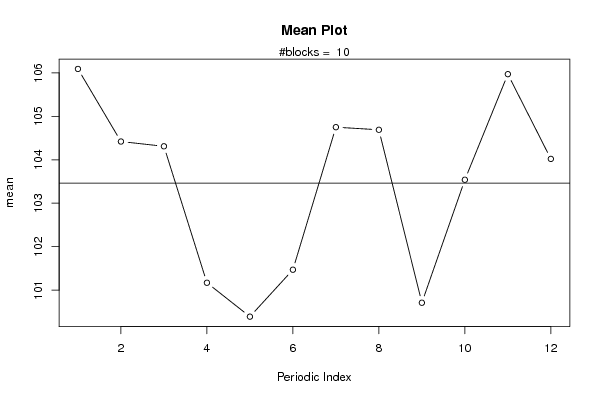PNG link Postscript link PDF link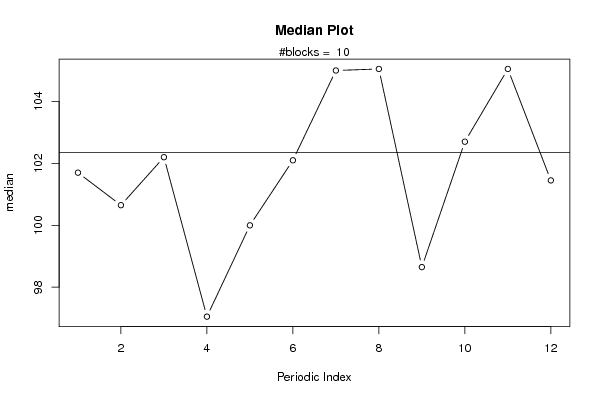PNG link Postscript link PDF linkPNG link Postscript link PDF link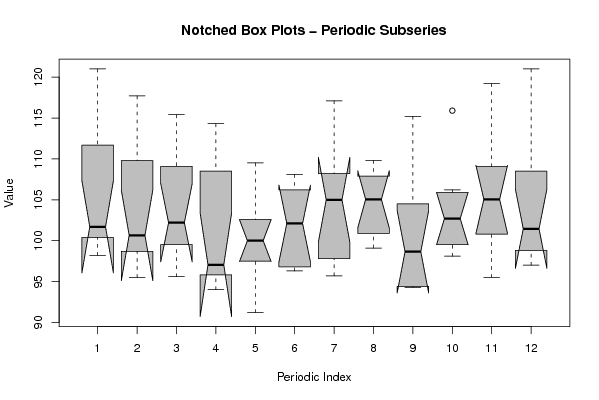PNG link Postscript link PDF link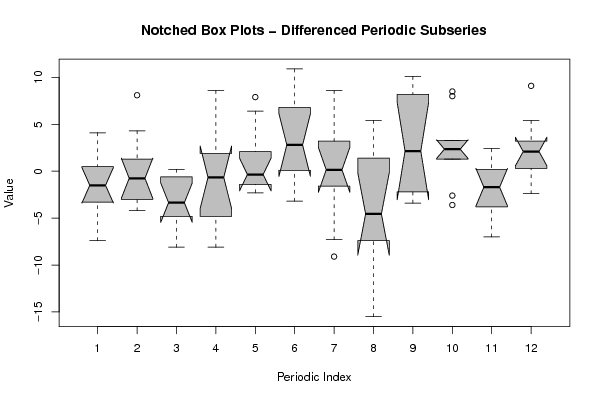PNG link Postscript link PDF link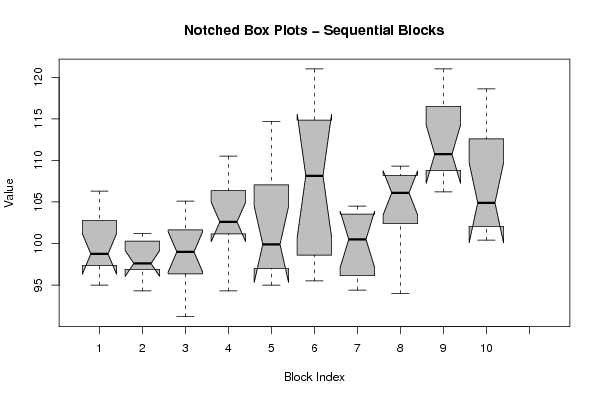PNG link Postscript link PDF link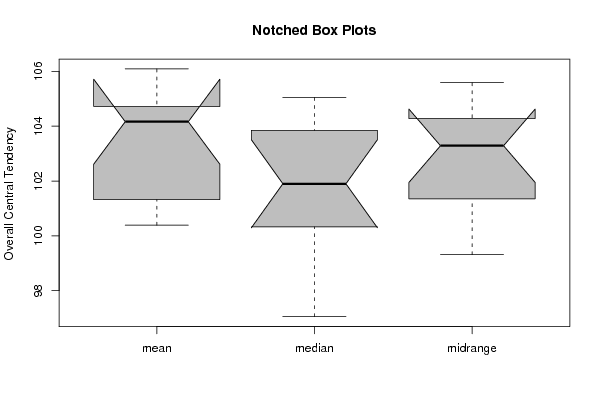PNG link Postscript link PDF link

Parameters (Session):
par1 = 12 ;
Parameters (R input):
par1 = 12 ;
R code (references can be found in the software module):
par1 <- as.numeric(par1)(n <- length(x))(np <- floor(n / par1))arr <- array(NA,dim=c(par1,np+1))darr <- array(NA,dim=c(par1,np+1))ari <- array(0,dim=par1)dx <- diff(x)j <- 0for (i in 1:n){j = j + 1ari[j] = ari[j] + 1arr[j,ari[j]] <- x[i]darr[j,ari[j]] <- dx[i]if (j == par1) j = 0}ariarrdarrarr.mean <- array(NA,dim=par1)arr.median <- array(NA,dim=par1)arr.midrange <- array(NA,dim=par1)for (j in 1:par1){arr.mean[j] <- mean(arr[j,],na.rm=TRUE)arr.median[j] <- median(arr[j,],na.rm=TRUE)arr.midrange[j] <- (quantile(arr[j,],0.75,na.rm=TRUE) + quantile(arr[j,],0.25,na.rm=TRUE)) / 2}overall.mean <- mean(x)overall.median <- median(x)overall.midrange <- (quantile(x,0.75) + quantile(x,0.25)) / 2bitmap(file='plot1.png')plot(arr.mean,type='b',ylab='mean',main='Mean Plot',xlab='Periodic Index')mtext(paste('#blocks = ',np))abline(overall.mean,0)dev.off()bitmap(file='plot2.png')plot(arr.median,type='b',ylab='median',main='Median Plot',xlab='Periodic Index')mtext(paste('#blocks = ',np))abline(overall.median,0)dev.off()bitmap(file='plot3.png')plot(arr.midrange,type='b',ylab='midrange',main='Midrange Plot',xlab='Periodic Index')mtext(paste('#blocks = ',np))abline(overall.midrange,0)dev.off()bitmap(file='plot4.png')z <- data.frame(t(arr))names(z) <- c(1:par1)(boxplot(z,notch=TRUE,col='grey',xlab='Periodic Index',ylab='Value',main='Notched Box Plots - Periodic Subseries'))dev.off()bitmap(file='plot4b.png')z <- data.frame(t(darr))names(z) <- c(1:par1)(boxplot(z,notch=TRUE,col='grey',xlab='Periodic Index',ylab='Value',main='Notched Box Plots - Differenced Periodic Subseries'))dev.off()bitmap(file='plot5.png')z <- data.frame(arr)names(z) <- c(1:np)(boxplot(z,notch=TRUE,col='grey',xlab='Block Index',ylab='Value',main='Notched Box Plots - Sequential Blocks'))dev.off()bitmap(file='plot6.png')z <- data.frame(cbind(arr.mean,arr.median,arr.midrange))names(z) <- list('mean','median','midrange')(boxplot(z,notch=TRUE,col='grey',ylab='Overall Central Tendency',main='Notched Box Plots'))dev.off()